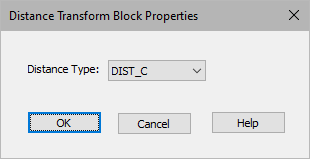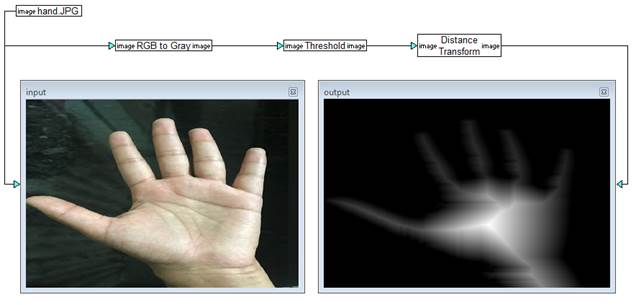### Distance Transform

Block Category: Operations

Input Image Requirements: Binary image

Description: Input to the Distance Transform block is a binary image with edges that are zero value and non-edges that are non-zero value. The Distance Transform block sets every output pixel to a value proportional to the distance to the nearest zero pixel in the input image. That is, the longer the distance to the nearest zero pixel, the brighter the intensity of the pixel.

The Distance Transform block uses a 3 x 3 masking array.#### Distance Type

DIST_C: Calculates the distance on a four-connected grid (horizontal and vertical).

DIST_L1: Calculates the distance on an eight-connected grid (horizontal, vertical, and diagonal).

DIST_L2: Calculates the exact Euclidean distances.

#### Example

In this example, DIST_L1 is applied to the input imaIn this example, DIST_L1 is applied to the input image.